# MATLAB Assignment 1

Math 222 Fall 2020
Question 1: Apply the Euler method to solvefrom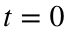to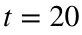.
Note that this problem has exact solutionwhich is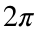-periodic. It is also easy to see how badly the method fails when you take N too large.
Then do the following:
• Figure out what is the minimum value of N that you need to get an error smaller than 0.01 at the final time. Do this by experimenting with N until you get a small enough error.
• Plot the computed and exact solution using this value of N.
• Show the method is approximately second order by doubling N and computing the ratio of the errors.
• Hint: MATLAB does not have the value of Euler's number e built in, but it does have the exponential function, i.e.t to evaluate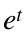, use the command exp(t), so that to get e, type exp(1).
I found that 1900 points was enough, but that 1890 was not. That's a lot!
tFinal = 20;
y0=1/2;
N = 1900;
h=tFinal/N;
t=linspace(0,tFinal,N+1); % type 'help linspace' to see how this works
y=zeros(1,N+1);y(1)=y0;
yX=yExact(t);
for n=1:N
y(n+1) = y(n) + h * f(t(n),y(n));
end
plot(t,y,t,yX,'--'); xlabel('t'); ylabel('y');
title('Result from Euler''s method');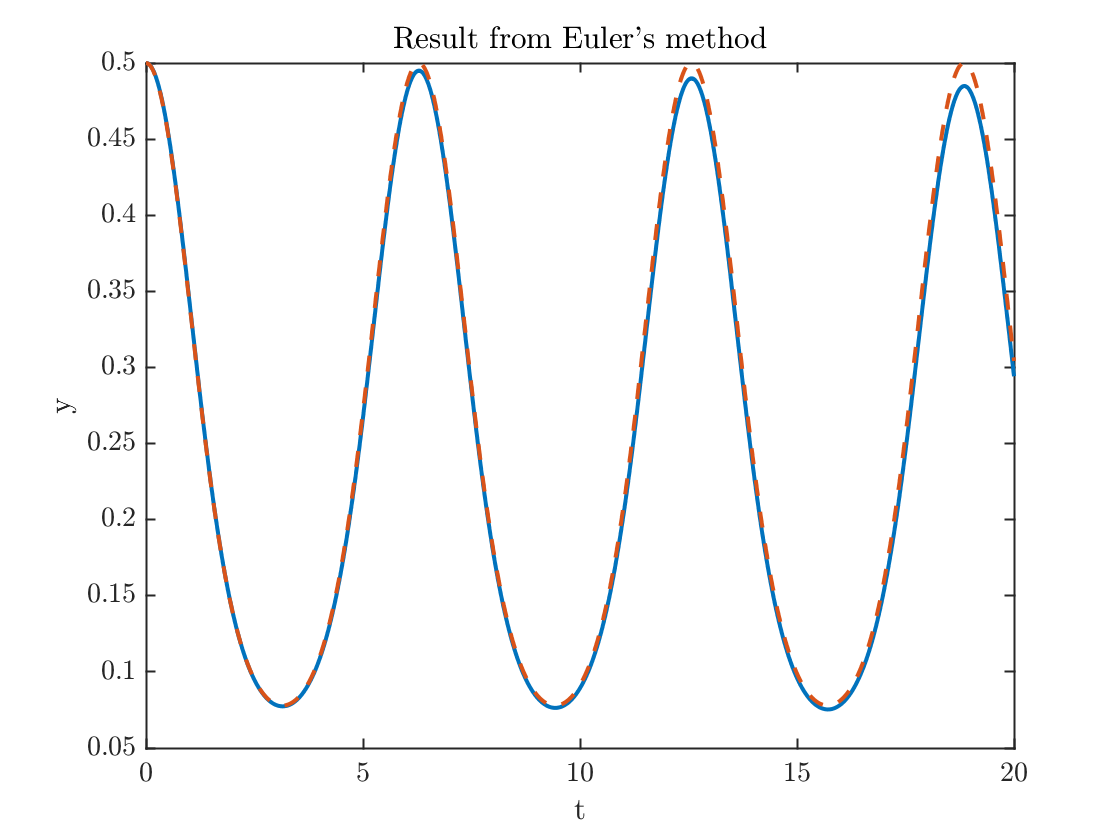plot(t,yX-y);xlabel('t');ylabel('error')
title('error from Euler''s method')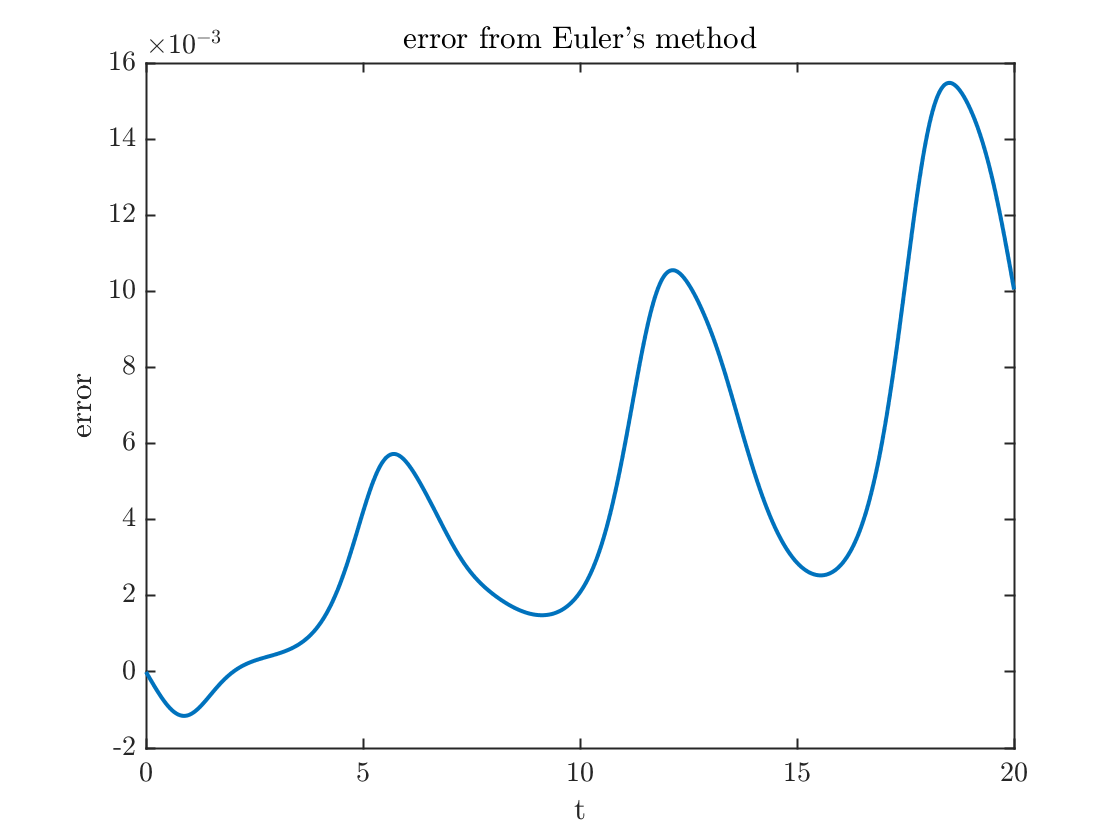error1= abs(y(N+1)-yX(N+1));
fprintf('The error at the final time = %0.4f.\n',error1)
The error at the final time = 0.0100.
Now I double the number of points and see that the error goes down by a factor of two.
N = 2*N;
h=tFinal/N;
t=linspace(0,tFinal,N+1); % type 'help linspace' to see how this works
y=zeros(1,N+1); y(1)=y0;
yX=yExact(t);
for n=1:N
y(n+1) = y(n) + h * f(t(n),y(n));
end
error2= abs(y(N+1)-yX(N+1));
fprintf('The error at the final time = %0.4f.\n',error2)
The error at the final time = 0.0050.
fprintf('The error went down by a factor of %f.\n',error1/error2);
The error went down by a factor of 1.996490.
Question 2 The Euler method is an example of a first order method. This means that the error is proportional to h, so that doubling the number of points roughly cuts the error roughly by a factor of two.
The improved Euler method is what's known as a two-step method. It requires two evaluations of the function per time step. At each step, we define two extra quantities before computing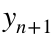.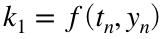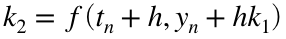The payoff for using this more complicated method is that it is second order! This means that each time you double the number of time steps the error goes down by a factor of four. This is a big deal.
Program this method to solve the same problem from Question 1 and
• Determine how large N needs to be get an error less than 0.01.
• Show the method is second order by doubling N and seeing that the error has gone down by a factor of four.
I found 50 points was enough to get the error below 0.01 at the final time but note that the error is worse when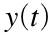is near its maximum value
N = 50;
h=tFinal/N;
t=linspace(0,tFinal,N+1); % type 'help linspace' to see how this works
y=zeros(1,N+1);y(1)=y0;
yX=yExact(t);
for n=1:N
k1=f(t(n),y(n));
k2=f(t(n)+h,y(n)+h*k1);
y(n+1) = y(n) + h * (k1+k2)/2;
end
plot(t,y,t,yX,'--'); xlabel('t'); ylabel('y');
title('Result from Improved Euler''s method');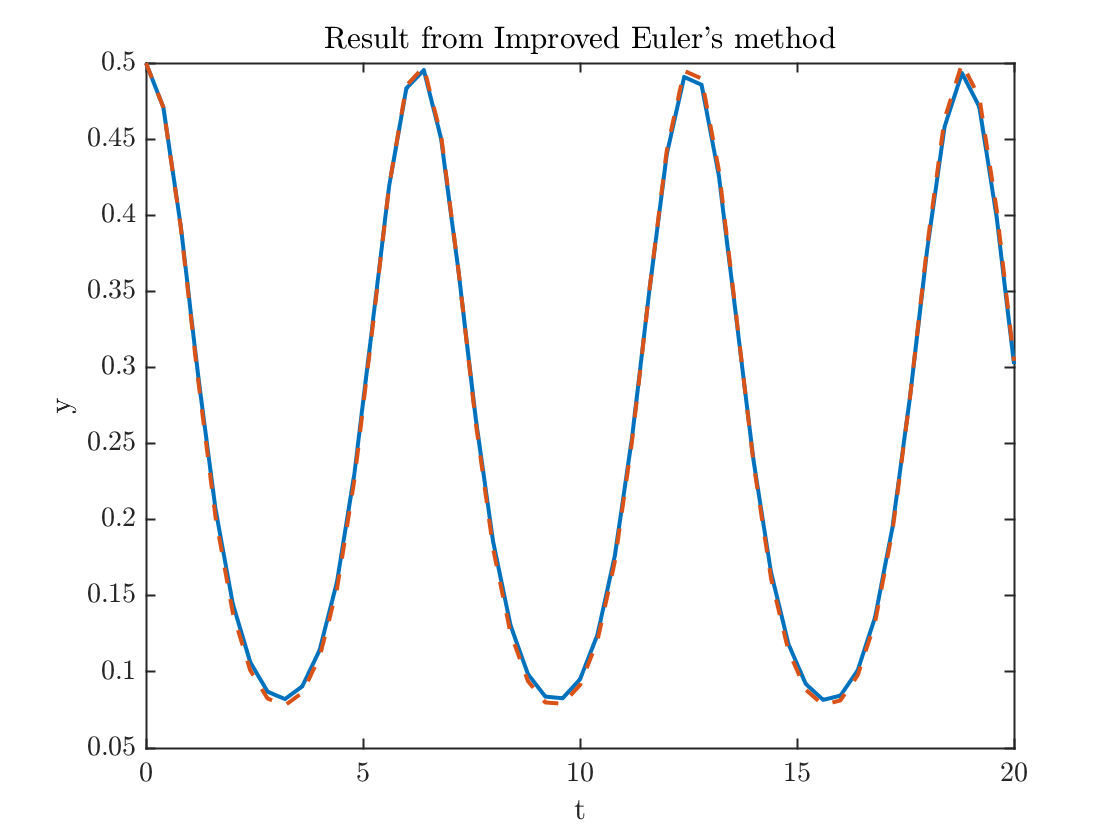plot(t,yX-y);xlabel('t');ylabel('error')
title('Error from Improved Euler''s method')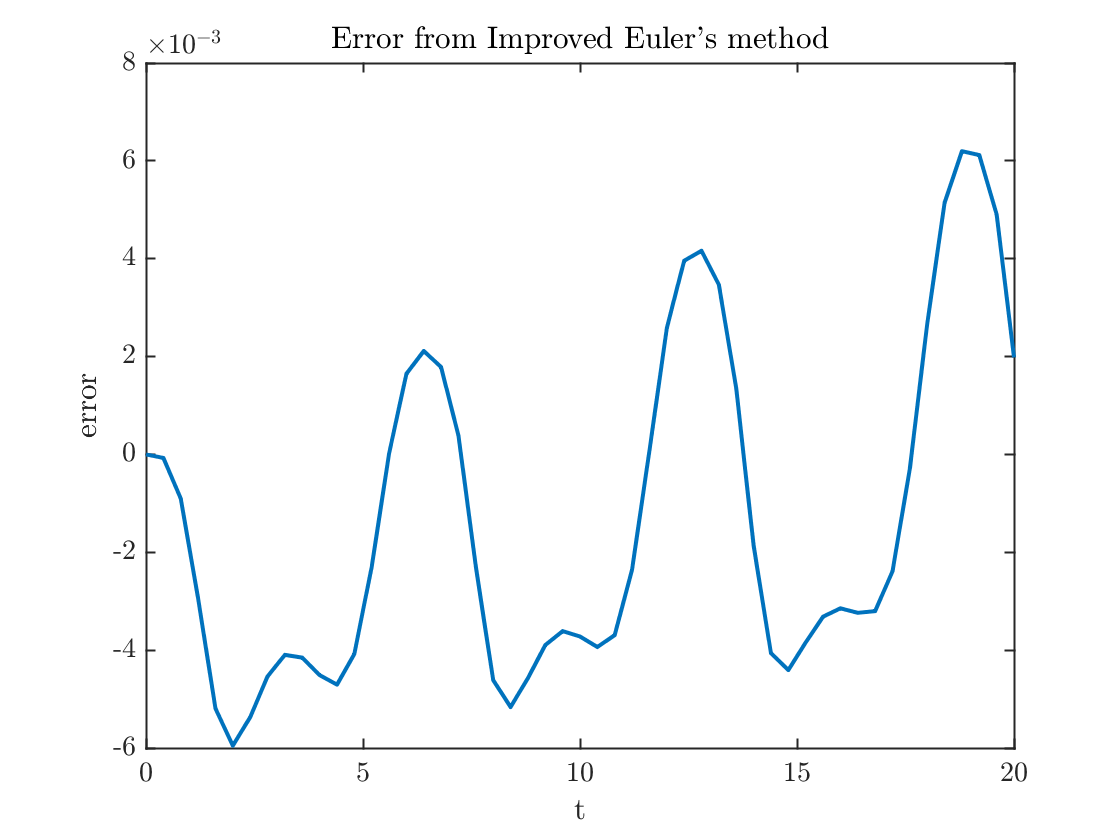error1= abs(y(N+1)-yX(N+1));
fprintf('The error at the final time = %0.4f.\n',error1)
The error at the final time = 0.0020.
In order to see the second order convergence, I need to use a larger value of N. Using M=1000 worked.
N = 1000;
h=tFinal/N;
t=linspace(0,tFinal,N+1); % type 'help linspace' to see how this works
y=zeros(1,N+1);y(1)=y0;
yX=yExact(t);
for n=1:N
k1=f(t(n),y(n));
k2=f(t(n)+h,y(n)+h*k1);
y(n+1) = y(n) + h * (k1+k2)/2;
end
error1= abs(y(N+1)-yX(N+1));
fprintf('The error at the final time = %0.df.\n',error1)
The error at the final time = 9e-06f.
N = 2*N;
h=tFinal/N;
t=linspace(0,tFinal,N+1); % type 'help linspace' to see how this works
y=zeros(1,N+1); y(1)=y0;
yX=yExact(t);
for n=1:N
k1=f(t(n),y(n));
k2=f(t(n)+h,y(n)+h*k1);
y(n+1) = y(n) + h * (k1+k2)/2;
end
error2= abs(y(N+1)-yX(N+1));
fprintf('The error at the final time = %0.df.\n',error2)
The error at the final time = 2e-06f.
fprintf('The error went down by a factor of %f.\n',error1/error2);
The error went down by a factor of 3.871636.
Question 3 In practice, scientists are most likely to use the fourth order Runge-Kutta method for time stepping. This requires four computations per step.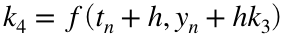The payoff for using this more complicated method is that it is fourth order! This means that each time you double the number of time steps the error goes down by a factor of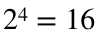. This is a really big deal.
Program this method to solve the same problem from Question 1 and
• Determine how large N needs to be get an error less than 0.01.
• Show the method is fourth order by doubling N and seeing that the error has gone down by a factor of sixteen.
For certain applications, such as computing spacecraft trajectories, scientists are likely to use methods that are of much higher order, even 12th order!
Remarkably, I was able to get the error below the desired level with only 18 points. This is fewer than I'd want to use in practice because, even if the numbers are good, what I really want is to know what the solution looks like, and this jagged plot doesn't really tell me. We say this computation is under-resolved.
N = 18;
h=tFinal/N;
t=linspace(0,tFinal,N+1); % type 'help linspace' to see how this works
y=zeros(1,N+1);y(1)=y0;
yX=yExact(t);
for n=1:N
k1=f(t(n),y(n));
k2=f(t(n)+h/2,y(n)+h*k1/2);
k3=f(t(n)+h/2,y(n)+h*k2/2);
k4=f(t(n)+h,y(n)+h*k3);
y(n+1) = y(n) + h * (k1+2*k2+2*k3+k4)/6;
end
plot(t,y,t,yX,'--'); xlabel('t'); ylabel('y');
title('Result from Runge-Kutta method');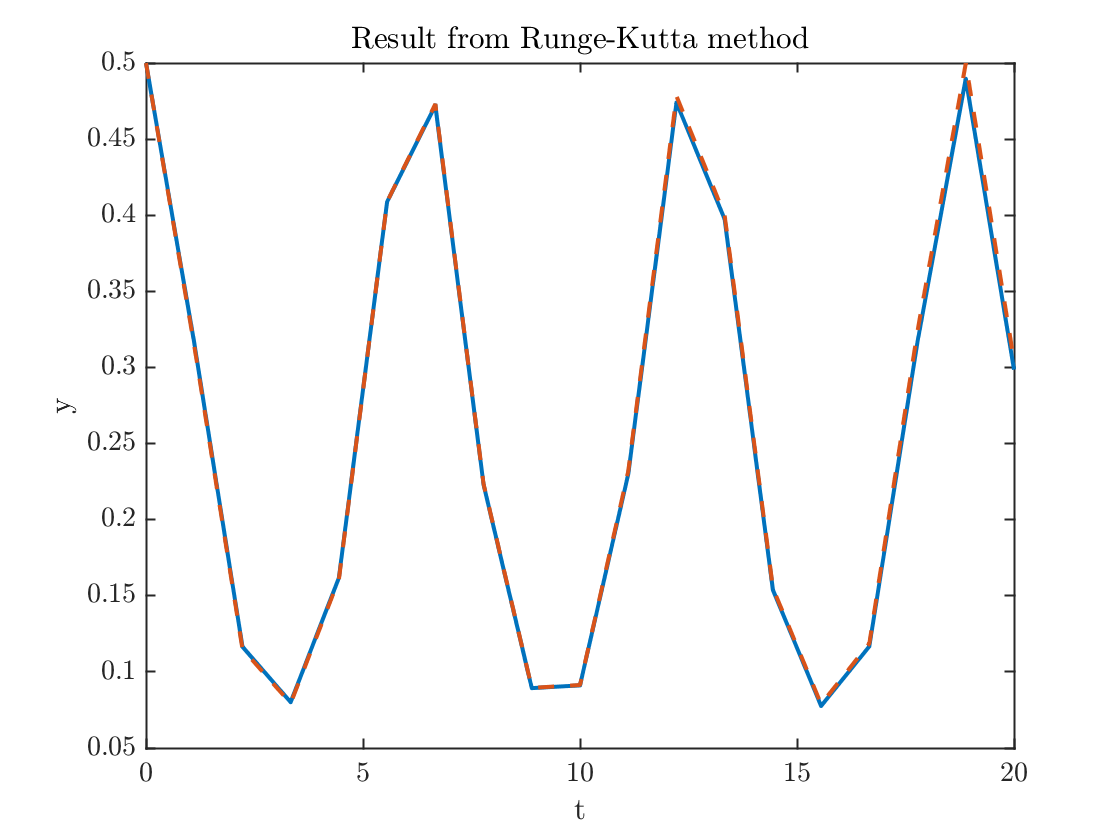plot(t,yX-y);xlabel('t');ylabel('error')
title('Error from Runge-Kutta method')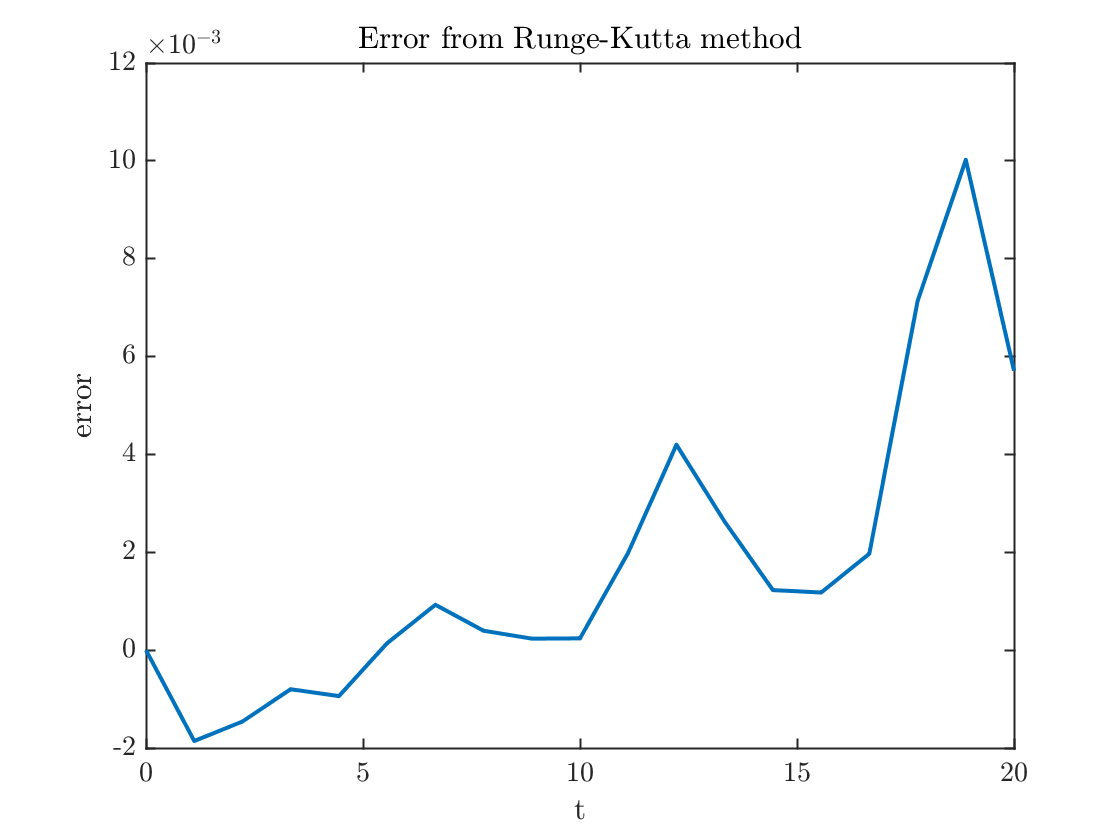error1= abs(y(N+1)-yX(N+1));
fprintf('The error at the final time = %0.4f.\n',error1)
The error at the final time = 0.0057.
In order to verify the fourth order convergence, I need to take N a lot smaller. Again I used N=1000.
N = 1000;
h=tFinal/N;
t=linspace(0,tFinal,N+1); % type 'help linspace' to see how this works
y=zeros(1,N+1);y(1)=y0;
yX=yExact(t);
for n=1:N
k1=f(t(n),y(n));
k2=f(t(n)+h/2,y(n)+h*k1/2);
k3=f(t(n)+h/2,y(n)+h*k2/2);
k4=f(t(n)+h,y(n)+h*k3);
y(n+1) = y(n) + h * (k1+2*k2+2*k3+k4)/6;
end
error1= abs(y(N+1)-yX(N+1));
fprintf('The error at the final time = %0.4d.\n',error1)
The error at the final time = 1.0447e-10.
Amazing, 10 digits of accuracy.
N = 2*N;
h=tFinal/N;
t=linspace(0,tFinal,N+1); % type 'help linspace' to see how this works
y=zeros(1,N+1); y(1)=y0;
yX=yExact(t);
for n=1:N
k1=f(t(n),y(n));
k2=f(t(n)+h/2,y(n)+h*k1/2);
k3=f(t(n)+h/2,y(n)+h*k2/2);
k4=f(t(n)+h,y(n)+h*k3);
y(n+1) = y(n) + h * (k1+2*k2+2*k3+k4)/6;
end
error2= abs(y(N+1)-yX(N+1));
fprintf('The error at the final time = %0.df.\n',error2)
The error at the final time = 7e-12f.
fprintf('The error went down by a factor of %f.\n',error1/error2);
The error went down by a factor of 15.577293.

## Functions used

function yprime=f(t,y)
yprime = (y^3-y)*sin(t);
end
function y=yExact(t)
y=exp(cos(t))./sqrt(3*exp(2)+exp(2*cos(t)));
end